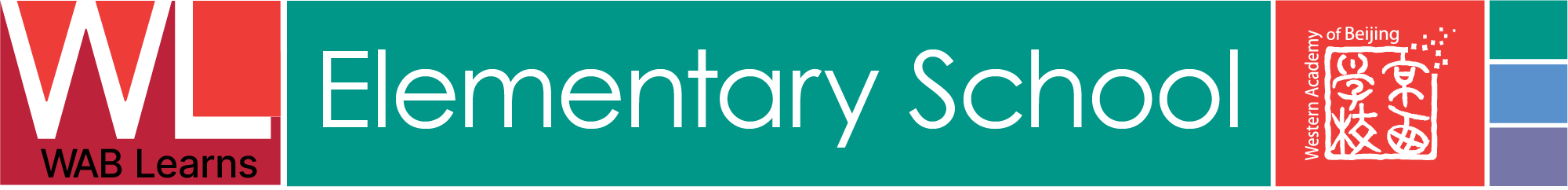## UNITS

Unit:
• Place Value up to 100,000
Essential Questions:
• How do we represent numbers up to 100,000?
• What are the different ways of writing numbers up to 100,000?
• What is the difference between expanded form and standard form?
• How can we use place value to order and compare numbers?
• How can we use place value to add and subtract numbers?
• How do we round numbers up to the nearest ten, hundred, thousand, and ten thousand?
• How can we use place value to multiply numbers?
• How can we use place value to divide numbers?
• What are the different strategies for solving two-digit by two-digit multiplication problems?
• How can we solve two-digit by two-digit division problems?
Unit:
• Addition and Subtraction: Mental and Written Strategies
Essential Questions:
• How can mental math strategies be used to solve addition and subtraction problems?
• What strategies can students use to solve addition and subtraction problems quickly and accurately?
• How do written strategies help students solve addition and subtraction problems?
• What strategies can students use to check their addition and subtraction solutions?
• When is it more efficient to use mental strategies versus written strategies to solve addition and subtraction problems?
• What strategies can students use to help them remember addition and subtraction facts?
Unit:
• Representing and Interpreting Data
Essential Questions:
• How can we use graphs and charts to represent data?
• How can we identify patterns in data?
• What is the difference between a bar graph, line graph, and a pictograph?
• How can we use data to draw conclusions?
• How can we interpret data accurately and effectively?
• What are the basic components of data analysis?
• How can we identify trends in data?
• How can data be used to make informed decisions?
• How can we make predictions based on data?
• How can we use data to compare and contrast information?
Unit:
• Problem Solving and Communicating
Essential Questions:
• How can students learn to work collaboratively when problem solving?
• How can students use mathematical language to explain their problem solving approaches?
• How do different strategies affect the outcomes of problem solving?
• How can problem solving help students become more independent learners?
• What strategies can students use to accurately assess their progress and understanding?
• What techniques can students use to promote effective communication when working on a problem?
• How can technology be used to enhance problem solving skills?
• How can teachers create learning environments that are conducive to the development of problem-solving skills?
Unit:
• Mass, Volume, and Capacity
Essential Questions:
• What is mass, volume, and capacity, and how do they differ from one another?
• How can we measure mass, volume, and capacity?
• How can we use these measurements to compare and order objects, and solve problems?
• How can we use mathematical reasoning to understand and solve problems related to mass, volume, and capacity?
• How can we use mathematical models to represent relationships between mass, volume, and capacity?
Unit:
• Multiplication and Division
Essential Questions:
• How can understanding multiplication and division help us solve real-world problems?
• How do multiplication and division relate to each other?
• What strategies can be used to solve multiplication and division problems efficiently and accurately?
• How can we use visuals to help us better understand multiplication and division?
• How does understanding multiplication and division help us understand other operations in mathematics?
Unit:
• Decimals and Fractions
Essential Questions:
• What is the connection between decimals and fractions?
• How can we compare and order fractions and decimals?
• What strategies can we use to add, subtract, multiply, and divide decimals and fractions?
• How can we use decimals and fractions to solve real-world problems?
• Why is it important to understand decimals and fractions?
Unit:
• 2D and 3D Shapes and Space
Essential Questions:
• How do 2D and 3D shapes differ?
• What relationships exist between shapes and the space they occupy?
• How can we use 2D and 3D figures to represent real-world objects and situations?
• How can we identify key features of 2D and 3D shapes?
• How can we use our understanding of shapes and space to solve problems?
• How can we visualize 3D shapes in a 2D context?
• How can we use symmetry to explore shapes and space?
• How can we measure and describe the properties of shapes and space?
Unit:
• Problem Solving and Reasoning
Essential Questions:
• What strategies can be used to solve a math problem?
• How can we use math to reason through a problem?
• What types of questions can be answered using problem solving and reasoning?
• How do we determine if our answer to a math problem is accurate?
• How can we use prior knowledge to think through a problem?
• What strategies can be used to check our work for accuracy?
• How do our answers to math problems help us to make real-world decisions?
• What strategies can be used to identify patterns in math problems?
• How can we use logic to solve a math problem?
• How can we use estimation to check our answer to a math problem?